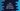# Generics in Dart explanation with example## Generics in Dart :

Generic is a concept to use different types of values with a single type of definition. For example, we can create different types of lists in dart like List is for a string list, List is for an integer list etc. Here, List is a generic type. If you check its definition, you will see that it is defined as List. Here, E letter stands for element. By convention letter E, T, S, K and V are used for generic. For example, map class is defined as Map<K, V>.Map, Set, List and Queue are examples of generic implementation.

In this tutorial, I will try to give you an idea of how generics work with different examples.

``````main(List<String> args) {
double avgInt = getAverage<int>(5, 6);
double avgDouble = getAverage<double>(5.9, 16.5);

print(avgInt);
print(avgDouble);
}

double getAverage<T extends num>(T first, T second) {
return (first + second) / 2;
}``````

### Example of generics (Generic method) :

Let’s consider the below example program :

Here, we have defined one method called getAverage to get an average of two values. It can take any type of parameter that extends num and returns one double as the response. Inside the main method, we are calculating the average of two integer values and two double values.

Using generic we can reduce the code duplication. Here, we are using type T as the parameter of a method. We can use the same type as its return value and also to create any other variables inside the method. Generic support was limited to classes initially in dart. Later, it was extended to use with methods as well.

### Example of generics with class (Generic class) :

Similar to the above example, we can also use generics with dart classes. Let’s consider the below example program :

``````class Property<T> {
T first, second;

Property(this.first, this.second);

String getDescription() {
return "First property : \${first}, Second property : \${second}";
}
}

main(List<String> args) {
var firstProperty = Property("Hello", 2);
var secondProperty = Property(2.30, 3.44);
var thirdProperty = Property(true, false);

print("firstProperty description \${firstProperty.getDescription()}");
print("secondProperty description \${secondProperty.getDescription()}");
print("thirdProperty description \${thirdProperty.getDescription()}");
}``````

Here, class Property takes two arguments in its constructor. These constructors can be of different types. In the main method, we are calling this constructor with string, double, integer and boolean. This works with all of these types. If you run this example, it will print the below output :

``````firstProperty description First property : Hello, Second property : 2
secondProperty description First property : 2.3, Second property : 3.44
thirdProperty description First property : true, Second property : false
``````